# Asian Option Pricing Formula for Uncertain Financial Market

## Abstract

Asian option is an important financial derivative instrument. It has been widely accepted by investors for its risk management property. Uncertain finance is a new field where the risk processes are described by uncertain processes. An asset price is assumed to follow a specific uncertain differential equation other than a stochastic differential equation. In this paper, Asian option models are proposed for uncertain financial market. Besides, Asian option pricing formulae are derived and some mathematical properties are investigated. Since the average price is presented in the Asian pricing formula which is difficult to compute, Yao-Chen formula is employed to solve this problem. Finally, several numerical examples are discussed.

## Introduction

The use of Brownian motion to evaluate stock options was first proposed by Bachelier in 1900. However, Brownian motion may take negative numbers, an alternative stochastic process geometric Brownian motion was employed in the pricing models, which started stochastic financial theory. In the early 1970s, Black and Scholes  and, independently, Merton  constructed the Black-Scholes formula to obtain the European option price with the proposed model. Since then, stock options played as an important risk management tool more and more accepted by investors. Meanwhile, European and American options were the two most used kinds of options. In 1987, Asian option was first introduced in Tokyo by a branch of an American bank where the payoff is determined by the average of underlying asset price over some pre-set period of time. This is different from the case of the usual European option and American option, where the payoff of the option contract depends on the price of the underlying instrument at or before exercise. There are two main advantages of Asian option such as: one is that it reduces the risk of market manipulation of the underlying instrument at maturity; another is that it has a cheeper price compared to European or American options for its the averaging feature reducing the volatility inherent in it. Asian options thus became one of the basic forms of exotic options. Asian option pricing has been studied by many researchers such as Kemna and Vorst  (using Monte Carlo techniques), Carverhill and Clewlow  (using Fourier transform method), Rogers and Shi  (transforming it into a parabolic PDE), and Geman and Yor  (using determined analysis). All these methods can be found in Ingersoll .

The above option pricing methods are developed from probability theory. As a different doctrine, we will study the Asian option pricing method based on uncertainty theory in this manuscript. Since probability distributions are always obtained from historical data, when no samples are available to estimate the probability distribution, we have to invite some domain experts to evaluate the degree of belief that each event will occur. In order to rationally deal with degree of belief, the uncertainty theory was founded by Liu  in 2007 as a branch of axiomatic mathematics for modeling human uncertainty. It has been subsequently studied by many researchers. Based on the assumption that stock price follows a geometric Liu process, uncertainty theory was first introduced into finance by Liu  in 2009. Furthermore, Liu  built an uncertain stock model and derived its European option price formulae. Chen  gave the American pricing formulae for Liu’s stock model. In addition, Peng and Yao  proposed a different uncertain stock model and derived the European and American option price formulae. Chen, Liu, and Ralescu  introduced an uncertain stock model with discrete dividends. Yu  studied an uncertain stock model with jumped. Chen and Gao  investigated the term structure for an uncertain interest rate model. Liu, Chen, and Ralescu  introduced the uncertain currency model and gave price formula for it. Besides, uncertainty theory was introduced to insurance models by Liu  based on the assumption that the claim process is an uncertain renewal reward process. Yao and Qin  built a new insurance risk process and derived the ruin index.

Different from European option and American option, the Asian option depends on the average price of the underlying asset. In order to get the average price, some properties of uncertain differential equations are needed. Chen and Liu  proved an existence and uniqueness theorem and gave a solution method for linear uncertain differential equations. However, only few types of uncertain differential equations have an analytic solution. In order to get numerical solutions, Yao and Chen  derived the Yao-Chen formula which relates uncertain differential equations and ordinary differential equations. In addition, Yao  derived the uncertainty distribution of integral of solutions to uncertain differential equations with respect to time. With the help of the theorems in Yao’s paper, we can get the price of the Asian option easily. For exploring the recent developments of the uncertainty theory, the readers may consult Liu . The main work of the manuscript is to introduce the Asian option for an uncertain financial market and to derive the Asian option pricing formulae for the proposed models. The rest of the paper is organized as follows. Some preliminary concepts of uncertain process are recalled in Section ‘Preliminary’. Uncertain stock models are recalled in Section ‘Uncertain stock models’. Asian call and put option pricing formulae are derived and some properties of them are discussed in Sections ‘Asian call option price’ and ‘Asian put option price’, respectively. Finally, a brief summary is given in Section ‘Conclusions’.

## Preliminary

Uncertainty theory is a branch of mathematics based on normality, duality, subadditivity, and product axioms. As a branch of axiomatic mathematics, uncertainty theory was founded by Liu  in 2007 and perfected by Liu  in 2009. The core content, uncertain measure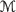, is defined as a set function on a non-empty set Γ satisfying the following axioms (Liu ): Axiom 1. (Normality Axiom)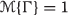for the universal set Γ; Axiom 2. (Duality Axiom)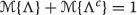for any event Λ; Axiom 3. (Subadditivity Axiom) For every countable sequence of events Λ 1,Λ 2,, we have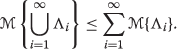The triplet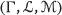is called an uncertainty space. The product uncertain measure was defined by Liu : Axiom 4. (Product Axiom) Let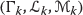be uncertainty spaces for k=1,2, Then the product uncertain measureis an uncertain measure satisfying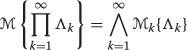where Λ k are arbitrarily chosen events from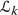for k=1,2,, respectively. Uncertain variable denoted by ξ is a measurable function from uncertain space to the set of real numbers. The inverse uncertainty distribution is frequently used to describe uncertain variables. Let ξ be an uncertain variable with an inverse uncertainty distribution Φ −1(α) (α(0,1)), if the expected value of ξ exists, then we have

$$E[\xi]={\int_{0}^{1}} \Phi^{-1}(\alpha)\mathrm{d} \alpha.$$

An uncertain process is essentially a sequence of uncertain variables indexed by time. The study of uncertain process was started by Liu  in 2008.

### Definition1.

(Liu ) Let T be an index set and letbe an uncertainty space. An uncertain process is a measurable function from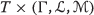to the set of real numbers, i.e., for each tT and any Borel set B of real numbers, the set

$$\{X_{t}\in B\}=\{\gamma\in \Gamma|X_{t}(\gamma)\in B\}$$

is an event.

An uncertain process X t is said to have independent increments if

$$X_{t_{0}}-X_{0}, X_{t_{1}}-X_{t_{0}}, X_{t_{2}}-X_{t_{1}},\cdots, X_{t_{k}}-X_{t_{k-1}}$$

are independent uncertain variables where t 0(≥0) is the initial time and t 1,t 2,,t k are any times with t 0<t 1<<t k . An uncertain process X t is said to have stationary increments if its increments are identically distributed uncertain variables whenever the time intervals have the same length, i.e., for any given t>0, the increments X s+t X s are identically distributed uncertain variables for all s>0. There is an important type of uncertain process called canonical Liu process with stationary and independent increments properties.

### Definition2.

(Liu ) An uncertain process C t is said to be a canonical Liu process if

1. (i)

C 0=0 and almost all sample paths are Lipschitz continuous,

2. (ii)

C t has stationary and independent increments,

3. (iii)

every increment C t+s C s is a normal uncertain variable with expected value 0 and variance t 2, whose uncertainty distribution is

$$\Phi(x)=\left(1+\exp\left(-\frac{\pi x}{\sqrt{3}t}\right)\right)^{-1},\ x\in\Re.$$

If C t is a canonical Liu process, then the uncertain process X t = exp(e t+σ C t ) is called a geometric Liu process. Based on the canonical Liu process, the Liu integral is introduced as follows.

### Definition3.

(Liu ) Let X t be an uncertain process and let C t be a canonical Liu process. For any partition of cloesd interval [a,b] with a=t 1<t 2<<t m+1=b, the mesh is written as

$$\triangle=\max\limits_{1\leq i\leq k}|t_{i+1}-t_{i}|.$$

Then the Liu integral of X t with respect to C t is

$${\int_{a}^{b}} X_{t}\mathrm{d} C_{t}=\lim\limits_{\triangle\rightarrow0}\sum_{i=1}^{k} X_{t_{i}}\cdot\left(C_{t_{i+1}}-C_{t_{i}}\right)$$

provided that the limit exists almost surely and is an uncertain variable.

Let h(t,c) be a continuously differentiable function. Then X t =h(t,C t ) is an uncertain process. Liu  proved the following chain rule

$$\mathrm{d} X_{t}=\frac{\partial h}{\partial t}(t,C_{t})\mathrm{d} t+\frac{\partial h}{\partial c}(t,C_{t})\mathrm{d} C_{t}.$$

Besides Liu integral, Yao integral was introduced by  which is a type of uncertain integral driven by uncertain renewal process. Chen  has also defined a type of uncertain integral driven by uncertain finite variation process.

An uncertain differential equation is essentially a type of differential equation driven by canonical Liu process.

### Definition4.

(Liu ) Suppose C t is a canonical Liu process,and f and g are two given functions.Then

$$\mathrm{d} X_{t}=f(t,X_{t})\mathrm{d} t+ g(t,X_{t})\mathrm{d} C_{t}$$

is called an uncertain differential equation. A solution is an uncertain process X t that satisfies the equation identically in t.

Chen and Liu  proved the existence and uniqueness theorem for uncertain differential equations. The solution method for solving linear uncertain differential equation was proposed by Chen and Liu . Besides, Liu  and Yao  proposed other solution methods for solving uncertain differential equations, respectively. However, most of the uncertain differential equations have no analytic solutions. Then finding numerical solution of the uncertain differential equation is particularly important. Yao-Chen formula relates uncertain differential equations and ordinary differential equations which proposes a numerical method for uncertain differential equations.

### Definition5.

(Yao and Chen ) Let αbe a number with 0<α<1. An uncertain differential equation

$$\mathrm{d} X_{t}=f(t,X_{t})\mathrm{d} t+ g(t,X_{t})\mathrm{d} C_{t}$$

is said to have an α-path $$X_{t}^{\alpha }$$ if it solves the corresponding ordinary differential equation

$$\mathrm{d} X_{t}^{\alpha}=f(t,X_{t})\mathrm{d} t+|g(t,X_{t})|\Phi^{-1}(\alpha)\mathrm{d} t$$
((1))

where Φ −1(α) is the inverse uncertainty distribution of standard normal uncertain variable,i.e.,

$$\Phi^{-1}(\alpha)=\frac{\sqrt{3}}{\pi} \ln \frac{\alpha}{1-\alpha}.$$

### Theorem1.

(Yao-Chen formula, Yao and Chen ) Let X t and $$X_{t}^{\alpha }$$ be the solution and α-path of the uncertain differential equation

$$\mathrm{d} X_{t}=f(t,X_{t})\mathrm{d} t+ g(t,X_{t})\mathrm{d} C_{t}$$

respectively. Then at each time t, the solution X t has an inverse uncertainty distribution

$$\Psi_{t}^{-1}(\alpha)=X_{t}^{\alpha}.$$

### Theorem2.

(Yao ) Let X t and $$X_{t}^{\alpha }$$ be the solution and α-path of the uncertain differential equation

$$\mathrm{d} X_{t}=f(t,X_{t})\mathrm{d} t+ g(t,X_{t})\mathrm{d} C_{t}$$

respectively. Assume J(x) is a strictly increasing function. Then the integral

$${\int_{0}^{s}} J(X_{t})\mathrm{d} t$$

has an inverse uncertainty distribution

$$\Psi^{-1}(\alpha)={\int_{0}^{s}} J\left(X_{t}^{\alpha}\right) \mathrm{d} t.$$

If J(x) is a strictly decreasing function, then the integral

$${\int_{0}^{s}} J(X_{t})\mathrm{d} t$$

has an inverse uncertainty distribution

$$\Psi^{-1}(\alpha)={\int_{0}^{s}} J\left(X_{t}^{1-\alpha}\right) \mathrm{d} t.$$

Yao and Chen  introduced the 99-method which is a numerical method for uncertain differential equations.

In order to calculate the uncertainty distribution of the integral of J(X t ) when J is a strictly increasing function, we have a numerical method as below. 99-method: Step 1: Fix α in (0,1), and fix h as the step length. Set i=0,N=s/h, and $$X_{0}^{\alpha }=X_{0}$$. Step 2: Employ the recursion formula

$$X_{i+1}^{\alpha}=X_{i}^{\alpha} + f\left(t,X_{i}^{\alpha}\right)h+g\left(t,X_{i}^{\alpha}\right)\Phi^{-1}(\alpha)h,$$

and calculate $$X_{i+1}^{\alpha }$$ and $$J\left (X_{i+1}^{\alpha }\right)$$. Step 3: Set ii+1. Step 4: Repeat Step 2 and Step 3 for N times. Step 5: The inverse uncertainty distribution of

$${\int_{0}^{s}} J(X_{t})\mathrm{d} t$$

is determined by

$$\Psi^{-1}_{s} (\alpha)=\sum_{i=1}^{N} J\left(X_{i}^{\alpha}\right)h.$$

We can use the 99-method to calculate the uncertainty distribution of $$X_{t}^{\alpha }$$ by taking J as identical. The numerical method is also quite the similar when J(x) is a strictly decreasing function.

## Uncertain stock models

Assumed that the stock price follows geometric Liu process, the first uncertain stock model was presented by Liu . In Liu’s stock model, the bond price X t and the stock price Y t are determined by

$$\begin{array}{@{}rcl@{}} \left\{ \begin{array}{l} \mathrm{d} X_{t}=rX_{t}\mathrm{d} t\\ \mathrm{d} Y_{t}=eX_{t}\mathrm{d} t+\sigma X_{t}\mathrm{d} C_{t} \end{array} \right. \end{array}$$
((2))

where r is the riskless interest rate, e is the stock drift, σ is the stock diffusion, and C t is a canonical Liu process.

Using the chain rule, we can easily find the analystic solution of the stock price

$$Y_{t}=Y_{0} \exp\left(et+\sigma C_{t}\right).$$

Based on uncertain stock model (2), the European option pricing formulas and the American option pricing formulas were derived by Liu  and Chen , respectively.

Another uncertain stock model was proposed by Peng and Yao  in which the stock price is assumed following mean-reversion uncertain differential equations. In Peng-Yao’s stock model, the bond price X t and the stock price Y t are determined by

$$\begin{array}{@{}rcl@{}} \left\{ \begin{array}{l} \mathrm{d} X_{t}=rX_{t}\mathrm{d} t\\ \mathrm{d} Y_{t}=(a-bY_{t})\mathrm{d} t+\sigma\mathrm{d} C_{t} \end{array} \right. \end{array}$$
((3))

where a, b and σ are constant and σ is the stock diffusion. Peng and Yao  also gave European formula and American option formula for this stock model. Besides, Chen, Liu, and Ralescu  proposed a stock model with dividends.

All the above stock models can be summarized up by a more general model where the bond price X t and the stock price Y t are determined by

$$\begin{array}{@{}rcl@{}} \left\{ \begin{array}{l} \mathrm{d} X_{t}=rX_{t}\mathrm{d} t\\ \mathrm{d} Y_{t}=f(t,Y_{t})\mathrm{d} t+g(t,Y_{t})\mathrm{d} C_{t} \end{array} \right. \end{array}$$
((4))

where r is the riskless interest rate,f and g are two functions and C t is a canonical Liu process. Based on the stock model (4), we will employ 99-method to get general formulae for Asian option pricing.

## Asian call option price

Consider the general uncertain stock model (4), we assume that the Asian call option has a strike price K and an expiration time T. If Y t is the price of the underlying stock, then the payoff of the Asian call option is defined by

$$P(T)=\left(\frac{1}{T} {\int_{0}^{T}} Y_{t} \mathrm{d} t-K \right)^{+}.$$

### Definition6.

Assume an Asian call option has a strike price K and an expiration time T. Then this option has price

$$f_{c}=\exp(-rT) E\left[\left(\frac{1}{T} {\int_{0}^{T}} Y_{t} \mathrm{d} t-K \right)^{+}\right].$$

### Theorem3.

Assume an Asian call option for the uncertain stock model (4) has an expiration time T and a strike price K. Then the Asian call option price is

$$f_{c}=\exp(-rT)\left(K\Psi_{T}(K)-K+\int_{\Psi_{T}(K)}^{1} \Psi_{T}^{-1}(\alpha) \mathrm{d} \alpha\right),$$
((5))

specifically,

$$\Psi_{T}^{-1}(\alpha)=\frac{1}{T} {\int_{0}^{T}} Y_{t}^{\alpha} \mathrm{d} t,$$

where $$Y_{t}^{\alpha }$$ stands for the α-path and α[0,1].

### Proof.

By the definition of Asian option, we have

$$f_{c}=\exp(-rT)E\left[\left(\frac{1}{T} {\int_{0}^{T}} Y_{t} \mathrm{d} t-K \right)^{+} \right].$$

Due to the definition of the expected value of uncertain variable, we have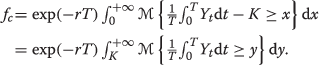Then we will use the integral of the solution of uncertain differential equation. It is obvious that x/T is a strictly increasing function of x. By Theorem 4, the integral

$$\frac{1}{T}{\int_{0}^{T}} Y_{t} \mathrm{d} t$$

has an inverse uncertainty distribution

$$\Psi_{T}^{-1}(\alpha)=\frac{1}{T} {\int_{0}^{T}} Y_{t}^{\alpha} \mathrm{d} t,$$
((6))

where $$Y_{t}^{\alpha }$$ is the α-path and α[0,1]. Again by the definition of the expected value of uncertain variable, and plug (6) into f c , we get

$$f_{c}=\exp(-rT)\left(K\Psi_{T}(K)-K+\int_{\Psi_{T}(K)}^{1} \Psi_{T}^{-1}(\alpha) \mathrm{d} \alpha\right).$$

### Remark.

It is obvious that the Asian call option formula of the general stock model (4) has the following properties: (i). f c is a decreasing function of the strike price K; (ii). f c is a decreasing function of the riskless interest rate r. As application of Asian call option pricing formula, we will consider three typical stock models under the following specific pre-set situations.

### Example1.

(Liu’s Stock Model) Consider Liu’s stock model. The α-path of equation is

$$Y_{t}^{\alpha}=Y_{0}\exp\left(\left(e+\sigma\Phi^{-1}(\alpha)\right)t\right)$$

where Y 0 is the initial value and Φ is a standard normal uncertainty distribution, i.e.

$$\Phi^{-1}(\alpha)=\frac{\sqrt{3}}{\pi} \ln \frac{\alpha}{1-\alpha}.$$

By the time-integral theorem, the average price $${\int _{0}^{T}} Y_{t} \mathrm {d} t/T$$ has an inverse uncertainty distribution

$$\begin{array}{@{}rcl@{}} \Psi_{T}^{-1}(\alpha)=\left\{ \begin{array}{cl} \frac{Y_{0}(\exp(e+\sigma\Phi^{-1}(\alpha)T)-1)}{T(e+\sigma\Phi^{-1}(\alpha))}, & \text{if}~\sigma\Phi^{-1}(\alpha)+e=0\\ Y_{0}, & \text{otherwise}. \end{array} \right. \end{array}$$
((7))

Using the Asian call option (5), we can easily obtain the Asian call option formula for Liu’s stock model.

### Example2.

In Peng-Yao’s stock model (3), the α-path of stock price Y t are determined by

$$\mathrm{d} Y_{t}^{\alpha}=\left((m-aY^{\alpha}_{t})+\sigma\Phi^{-1}(\alpha)\right) \mathrm{d} t.$$

The solution of the above equation is

$$Y_{t}^{\alpha}=Y_{0}\exp (-at)+ (1-\exp(-at))\left(\frac{m}{a}+\frac{\sigma}{a} \Phi^{-1}(\alpha)\right),$$

where Y 0 is a initial price and Φ is the standard normal uncertainty distribution, i.e.

$$\Phi^{-1}(\alpha)=\frac{\sqrt{3}}{\pi} \ln \frac{\alpha}{1-\alpha}.$$

By the time-integral theorem, the average price $${\int _{0}^{T}} Y_{t} \mathrm {d} t$$ has an inverse uncertainty distribution

$$\Psi_{T}^{-1}(\alpha)=T\left(\frac{m}{a}+\frac{\sigma}{a}\Phi^{-1}(\alpha)\right) +\left(\frac{m}{a^{2}}+\frac{\sigma}{a^{2}}\Phi^{-1}(\alpha)-\frac{1}{a}\right)(\exp(-at)-1).$$

Plug $$\Psi _{T}^{-1}(\alpha)$$ into the Asian call option pricing formula (5), we can get the Asian option price.

### Example3.

Consider another stock model, in which the bond price X t and the stock price Y t are determined by

$$\begin{array}{@{}rcl@{}} \left\{ \begin{array}{l} \mathrm{d} X_{t}=rX_{t}\mathrm{d} t\\ \mathrm{d} Y_{t}=(m-aY_{t})\mathrm{d} t+\sigma \sqrt{Y_{t}}\mathrm{d} C_{t} \end{array} \right. \end{array}$$
((8))

where r is the riskless interest rate, m and a are two positive coefficients, σ is the stock diffusion, and C t is a canonical Liu process. The analysic solution of Y t does not exist. Then we will use Yao-Chen formula and 99-method to get the inverse uncertainty distribution of $$Y_{t}^{\alpha }$$, thus get the inverse uncertainty distribution Ψ −1(α) of the average price $${\int _{0}^{T}} Y_{t} \mathrm {d} t$$ by the integral theorem. Plug Ψ −1(α) into the Asian call option pricing formula so that we can get the Asian option price.

## Asian put option price

Consider the general uncertain stock model, we assume that the Asian put option has strike price K and expiration time T. If Y t is the price of the underlying stock, then the payoff of the Asian put option is defined by

$$P(T)=\left(K-\frac{1}{T} {\int_{0}^{T}} Y_{t} \mathrm{d} t \right)^{+}.$$

### Definition7.

Assume an Asian put option has a strike price K and an expiration time T. Then this option has price

$$f_{p}=E\left[\exp(-rT) \left(K-\frac{1}{T} {\int_{0}^{T}} Y_{t} \mathrm{d} t \right)^{+}\right].$$

### Theorem4.

Assume the Asian call option for the general uncertain stock model has an expiration time T and a strike price K. Then the Asian put option price is

$$f_{p}=\exp(-rT)\left(K\Psi_{T}(K)-\int_{\Psi_{T}(0)}^{\Psi(K)} \Psi_{T}^{-1}(\alpha) \mathrm{d} \alpha \right)$$
((9))

specifically,

$$\Psi_{T}^{-1}(\alpha)=\frac{1}{T} {\int_{0}^{T}} Y_{t}^{\alpha} \mathrm{d} t,$$

where $$Y_{t}^{\alpha }$$ is the α-path and α[0,1].

### Proof.

By the definition of Asian option, we have

$$f_{p}=\exp(-rT)E\left[\left(K-\frac{1}{T} {\int_{0}^{T}} Y_{t} \mathrm{d} t \right)^{+} \right]$$

Due to the definition of the expected value of uncertain variable, we have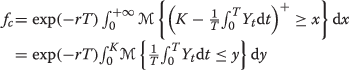Then we will use the integral of the solution of uncertain differential equation. It is obvious that x/T is a strictly increasing function of x. By Theorem 4, the integral

$$\frac{1}{T}{\int_{0}^{T}} Y_{t} \mathrm{d} t$$

has an inverse uncertainty distribution

$$\Psi_{T}^{-1}(\alpha)=\frac{1}{T} {\int_{0}^{T}} Y_{t}^{\alpha} \mathrm{d} t,$$

where $$Y_{t}^{\alpha }$$ is the α-path of and α[0,1]. Again by the definition of the expected value of uncertain variable, and plug 3.4 into f p , we get

$$f_{p}=\exp(-rT)\left(K\Psi_{T}(K)-\int_{\Psi_{T}(0)}^{\Psi(K)} \Psi_{T}^{-1}(\alpha) \mathrm{d} \alpha \right).$$

### Remark.

It is obvious that the Asian put option formula of the general stock model (4) has the following properties: (i). f p is an increasing function of the strike price K; (ii). f p is a decreasing function of the riskless interest rate r.

Just as what we have done in Section ‘Asian call option price’, we will consider three typical stock models under the following specific pre-set situation as an application of the put option pricing formula.

### Example4.

(Liu’s Stock Model) Consider Liu’s stock model. The α-path of equation is

$$Y_{t}^{\alpha}=Y_{0}\exp\left(\left(e+\sigma\Phi^{-1}(\alpha)\right)t\right)$$

where Y 0 is the initial value and Φ is a standard normal uncertainty distribution, i.e.

$$\Phi^{-1}(\alpha)=\frac{\sqrt{3}}{\pi} \ln \frac{\alpha}{1-\alpha}.$$

By the time-integral theorem, the average price $${\int _{0}^{T}} Y_{t} \mathrm {d} t/T$$ has an inverse uncertainty distribution

$$\begin{array}{@{}rcl@{}} \Psi_{T}^{-1}(\alpha)=\left\{ \begin{array}{cl} \frac{Y_{0}(\exp(e+\sigma\Phi^{-1}(\alpha)T)-1)}{T(e+\sigma\Phi^{-1}(\alpha))}, & \text{if}~ \sigma\Phi^{-1}(\alpha)+e=0\\ Y_{0}, & \text{otherwise}. \end{array} \right. \end{array}$$
((10))

Using the Asian put option (9), we can easily obtain the Asian call option formula for Liu’s stock model.

### Example5.

In Peng-Yao’s stock model (3), the α-path of stock price Y t are determined by

$$\mathrm{d} Y_{t}^{\alpha}=\left((m-aY^{\alpha}_{t})+\sigma\Phi^{-1}(\alpha)\right) \mathrm{d} t.$$

The solution of the above equation is

$$Y_{t}^{\alpha}=Y_{0}\exp (-at)+ (1-\exp(-at))\left(\frac{m}{a}+\frac{\sigma}{a} \Phi^{-1}(\alpha)\right),$$

where Y 0 is a initial price and Φ is the standard normal uncertainty distribution, i.e.

$$\Phi^{-1}(\alpha)=\frac{\sqrt{3}}{\pi} \ln \frac{\alpha}{1-\alpha}.$$

By the time-integral theorem, the average price $${\int _{0}^{T}} Y_{t} \mathrm {d} t$$ has an inverse uncertainty distribution

$$\Psi_{T}^{-1}(\alpha)=T\left(\frac{m}{a}+\frac{\sigma}{a}\Phi^{-1}(\alpha)\right)+\left(\frac{m}{a^{2}} +\frac{\sigma}{a^{2}}\Phi^{-1}(\alpha)-\frac{1}{a}\right)(\exp(-at)-1).$$

Plug $$\Psi _{T}^{-1}(\alpha)$$ into the Asian put option pricing formula (9), we can get the Asian option price.

### Example6.

Consider another stock model (8), in which the analysic solution of Y t does not exist. Then we will use Yao-Chen formula and 99-method to get the inverse uncertainty distribution of $$Y_{t}^{\alpha }$$, thus get the inverse uncertainty distribution Ψ −1(α) of the average price $${\int _{0}^{T}} Y_{t} \mathrm {d} t/T$$ by the integral theorem. Plug Ψ −1(α) into the Asian put option pricing formula so that we can get the Asian option price.

## Conclusions

In this paper, Asian options for uncertain market was proposed. Besides, Asian call and put option pricing formulae were obtained for uncertain stock models via Yao-Chen formula. Some mathematical properties of these formulas were also studied. Asian option pricing formulae for three uncertain stock models were calculated, respectively.

## References

1. Black, F, Scholes, M: The pricing of option and corporate liabilities. J. Polit. Econ. 81, 637–654 (1973).

2. Carverhill, A, Clewlow, L: Flexible convolution. In: From Black Scholes to Black Holes- New Frontiers in Options, pp. 165–171. Risk Magazine, London (1992).

3. Chen, X, Liu, B: Existence and uniqueness theorem for uncertain differential equations. Fuzzy Optim. Decis. Making. 9(1), 69–81 (2010).

4. Chen, X: American option pricing formula for uncertain financial market. Int. J. Oper. Res. 8(2), 32–37 (2011).

5. Chen, X, Liu, Y, Ralescu, DA: Uncertain stock model with periodic dividends. Fuzzy Optim. Decis. Making. 12(1), 111–123 (2013).

6. Chen, X: Uncertain calculus with finite variation processes. Soft. Comput. (2015). doi:10.1007/s00500-014-1452-0.

7. Chen, X, Gao, J: Uncertain term structure model of interest rate. Soft. Comput. 17(4), 597–604 (2013).

8. Geman, H, Yor, M: Bessel processes, Asian options and perpetuities. Math. Financ. 3, 349–375 (1993).

9. Ingersoll, J: Theory of financial decision making. Blackwell, Oxford (1987).

10. Kemna, A, Vorst, A: A pricing method for options based on average asset values. J. Bank Financ. 14, 113–129 (1990).

11. Liu, B: Uncertainty theory. 2nd ed. Springer-Verlag, Berlin (2007).

12. Liu, B: Fuzzy process, hybrid process and uncertain process. J. Uncertain Sys. 2(1), 3–16 (2008).

13. Liu, B: Some research problems in uncertainty theory. J. Uncertain Sys. 3(1), 3–10 (2009).

14. Liu, B: Uncertainty theory: a branch of mathematics for modeling human uncertainty. Springer-Verlag, Berlin (2010).

15. Liu, B: Extreme value theorems of uncertain process with application to insurance risk model. Soft. Comput. 17(4), 549–556 (2013).

16. Liu, Y: An analytic method for solving uncertain differential equations. J. Uncertain Sys. 6(4), 244–249 (2012).

17. Liu, Y, Chen, X, Dan, A: Ralescu, Uncertain currency model and currency option pricing. Int. J. Intell. Syst. 30(1), 40–51 (2015).

18. Merton, R: Theory of rational option pricing. Bell J. Econ. Manag. Sci. 4(1), 141–183 (1973).

19. Peng, J, Yao, K: A new option pricing model for stocks in uncertainty markets. Int. J. Oper. Res. 8(2), 18–26 (2011).

20. Rogers, L, Shi, Z: The value of an Asian option. J. Appl. Probab. 32, 1077–1088 (1995).

21. Yao, K: Uncertain calculus with renewal process. Fuzzy Optim. Decis. Making. 11(3), 285–297 (2012).

22. Yao, K: Extreme values and integral of solution of uncertain differential equation. J. Uncertain. Anal. Appl. 1, 3 (2013).

23. Yao, K, Chen, X: A numerical method for solving uncertain differential equations. J. Intell. Fuzzy Syst. 25(3), 825–832 (2013).

24. Yao, K: A type of uncertain differential equations with analytic solution. J. Uncertainty Anal. Appl. 1, 8 (2013).

25. Yao, K, Qin, Z: A modified insurance risk process with uncertainty. Insur. Math. Econ. 62, 227–233 (2015).

26. Yu, X: A stock model with jumps for uncertain markets. Int. J. Uncertaint. Fuzziness and Knowl.-Based Syst. 20(3), 421–432 (2012).

## Acknowledgements

This work was supported by National Natural Science Foundation of China Grant No.61273044 and No.61304182.

## Author information

Authors

### Corresponding author

Correspondence to Xiaowei Chen.

## Rights and permissions